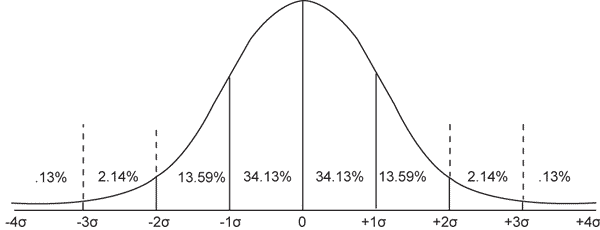SEARCH HOMEMath Central Quandaries & QueriesQuestion from ADRIAN, a student: Here is my question. Can you help? If you are told that the mean salary of a certain group of workers is $30,000 with a standard deviation of$4000, what proportion of workers earn over $38,000? What proportion of workers earn less than$18,000? Assume the distribution of wages is normal."Assume the distribution of wages is normal" means that it follows a normal distribution curve (this used to be called a bell curve because of its shape). This is a very well-defined curve that has some specific properties relating to area.The percentages are the proportion of the area under each portion of the curve compared to the area under the entire curve. This means that the entire group of salaries is the area under the curve, the 0 point is the mean salary and σ is the standard deviation.

Here's an example I'll solve:

Question: If the mean time for a hard drive to fail is 4 years and the standard deviation is 9 months, then what is the chance that the hard drive will last longer than 5½ years?

Solution: 5½ years is 1½ years away from the mean. 1½ years is twice the standard deviation of 9 months. So we are talking about the area under the curve to the right of the +2σ line. That is 2.27% (because we add 2.14% to 0.13%).

Hope this helps you with your problem,
Stephen La Rocque.Math Central is supported by the University of Regina and The Pacific Institute for the Mathematical Sciences.